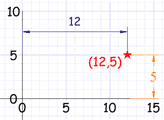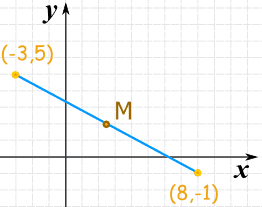# Midpoint of a Line Segment

### Coordinate PointHere the point (12,5) is
12 units along, and 5 units up

We can use Cartesian Coordinates to locate a point by how far along and how far up it is:

And when we know both end points of a line segment we can find the midpoint "M" (try dragging the blue circles):

## Midpoint of a Line SegmentThe midpoint is halfway between the two end points:

• Its x value is halfway between the two x values
• Its y value is halfway between the two y values

To calculate it:

• Add both "x" coordinates, divide by 2
• Add both "y" coordinates, divide by 2As a formula:

M = ( xA+xB 2 , yA+yB 2 )

### Example: What is the midpoint here?Use the formula:

M = ( xA+xB 2 , yA+yB 2 )

M = ( (−3)+8 2 , 5+(−1) 2 )

M = ( 5/2, 4/2 )

M = ( 2.5, 2 )